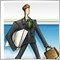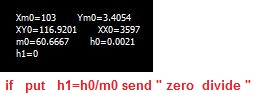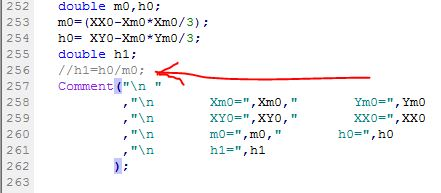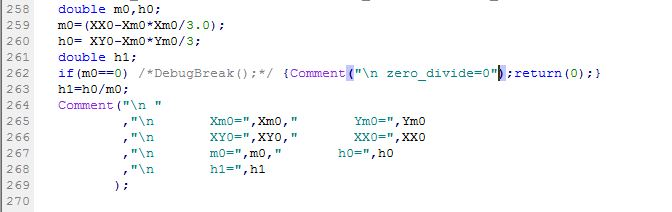# send " zero divide " but its not. why ?4796

hi.

in attached code if put   "   h1=h0/m0;   "  as same as in code, then send  " zero  divide ".where is the problem.?

Files:38676

Isn't obvious that m0 = 0 will give you a zero divide error ?4796

Alain Verleyen:
Isn't obvious that m0 = 0 will give you a zero divide error ?

m0 is not equal zero.38676

m0 is not equal zero.

Yes it is.4796

Alain Verleyen:

Yes it is.

thank you . HAVE good new year 2019that's work.

thank you.